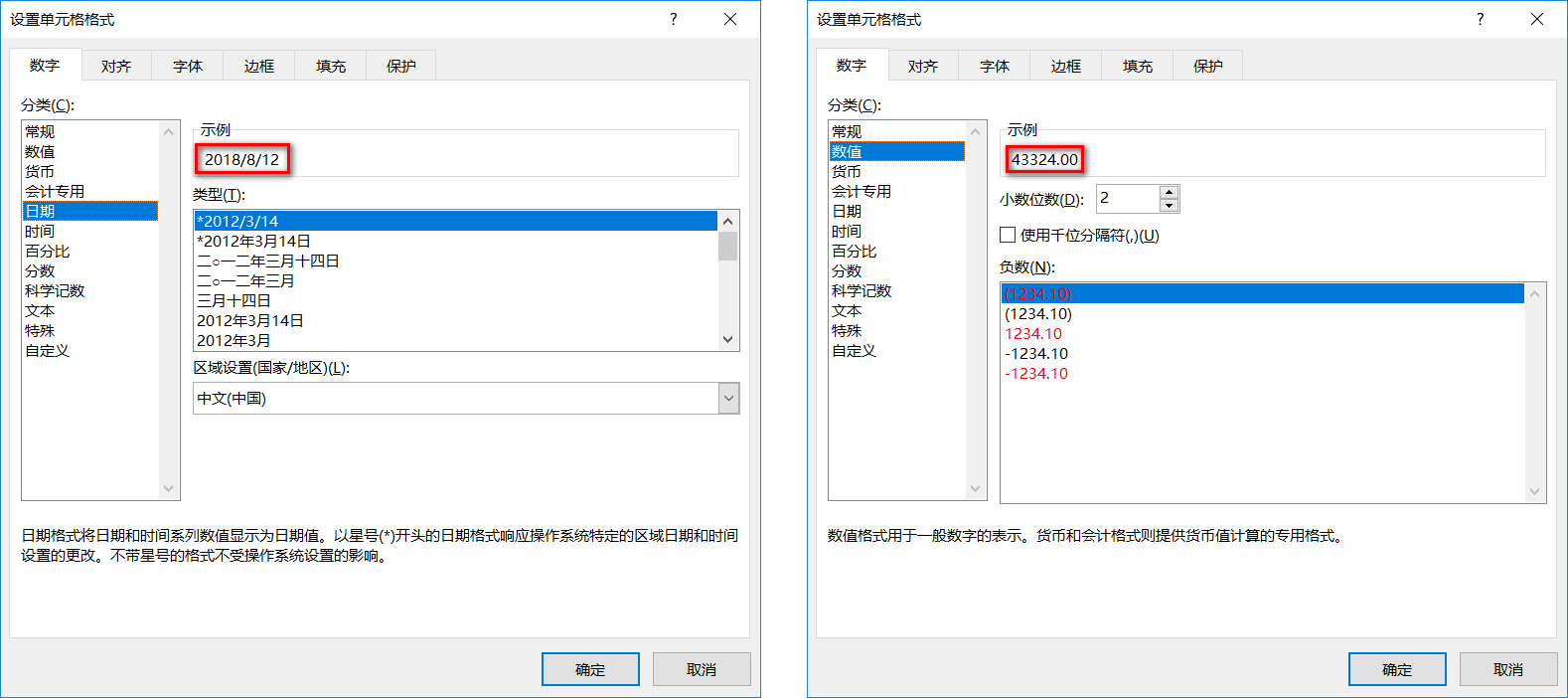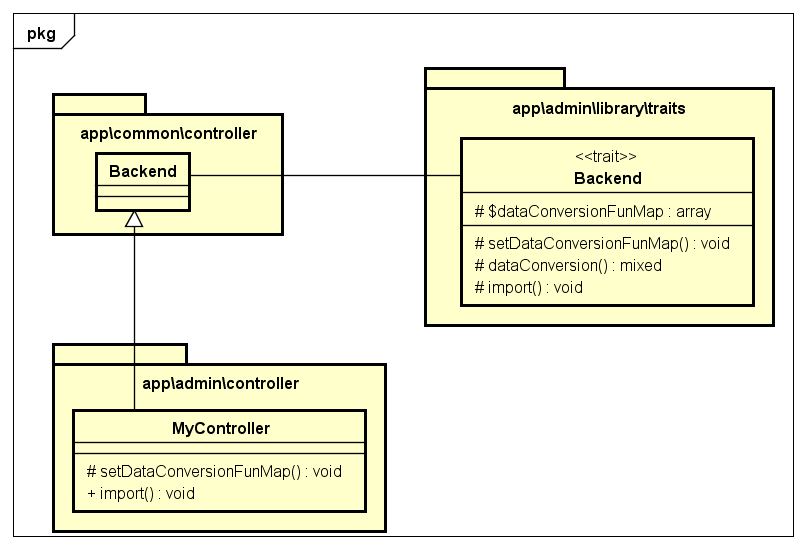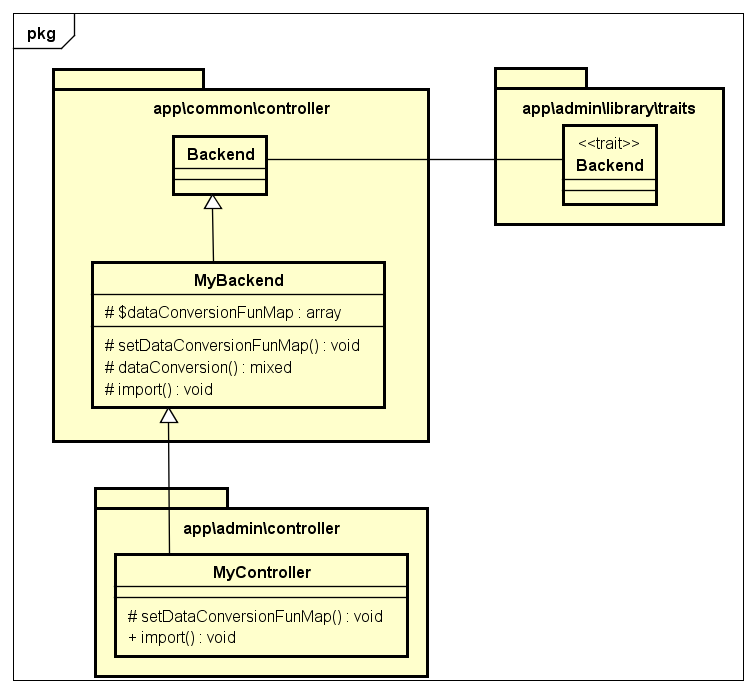## 日期导入出错问题的原因和解决办法

### 日期导入出错问题的原因

Excel中的日期和时间数据是以数值格式存储的，例如日期2018/8/12，实际上是以数值43324存储的。### 日期导入出错问题的解决办法

``````<?php
date("Y-m-d", \PHPExcel_Shared_Date::ExcelToPHP(43324));
/* 2018/8/12 */
``````

``````/*
* 字段-数据转换函数 键值对数组
*/
protected \$dataConversionFunMap = [];
``````
``````/*
* 设置 字段-数据转换函数 键值对数组
*/
protected function setDataConversionFunMap()
{
\$this->dataConversionFunMap = [];
}

/*
* 数据转换
*/
private function dataConversion(\$fieldArr, \$k, \$v)
{
\$this->setDataConversionFunMap();
if (isset(\$this->dataConversionFunMap)) {
foreach (\$this->dataConversionFunMap as \$field => \$dataConversionFun) {
if (\$fieldArr[\$k] === \$field) {
return \$dataConversionFun(\$v);
} else {
return \$v;
}
}
} else {
return \$v;
}
}
``````
1. 修改\app\admin\library\traits\Backend.php的import()方法。将其中的`\$row[\$fieldArr[\$k]] = \$v;`这一行改成`\$row[\$fieldArr[\$k]] = \$this->dataConversion(\$fieldArr, \$k, \$v);`
``````// 修改前
foreach (\$temp as \$k => \$v) {
if (isset(\$fieldArr[\$k]) && \$k !== '') {
\$row[\$fieldArr[\$k]] = \$v;
}
}

// 修改后
foreach (\$temp as \$k => \$v) {
if (isset(\$fieldArr[\$k]) && \$k !== '') {
\$row[\$fieldArr[\$k]] = \$this->dataConversion(\$fieldArr, \$k, \$v);
}
}
``````
1. 如何使用？
在自己的控制器中重写setDataConversionFunMap()方法。
假设要进行数据转换的字段为warehousing_date，将它和针对它进行数据转换处理的函数加入键值对数组。
这样导入Excel表时，我们设置的数据转换函数就会对字段为warehousing_date的数据进行处理了。
``````/*
* 设置 字段-数据转换函数 键值对数组
*/
protected function setDataConversionFunMap()
{
\$dataConversionFunMap['warehousing_date'] = function (\$v) {
if(\$v){
return date("Y-m-d", \PHPExcel_Shared_Date::ExcelToPHP(\$v));
}
return date('Y-m-d');
};
\$this->dataConversionFunMap = \$dataConversionFunMap;
}
``````

### 后续思考

UML类图